# 9.7   Data Analysis

This section under major construction.

Normal distribution. Sum of a bunch of random coin flips.

Random sampling. Physical measurement of some unknown constant, e.g., gravitational constant. There is some error associated with each measurement, so we get slightly different results each time. Our goal is to estimate the unknown quantity as accurately and precisely as possible. The sample mean and sample variance are defined as: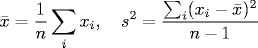The sample mean estimates the unknown constant, and the sample variance measures how precise the estimate is. Under quite general conditions, as n gets large, the sample mean obeys a normal distribution with mean equal to the unknown constant and variance equal to the the sample variance. The 95% approximate confidence interval isThe confidence interval measures the uncertainty assoicated with our estimate of the unknown constant. It means that if we perform the same experiment many times, we would expect that 95% of the time the estimated mean would lie in the given interval. The number 1.96 occurs because the probability that a normal random variable is between -1.96 and 1.96 happen to be 95%. If we want a 90% or 99% confidence interval, substitute 1.645 or 2.575, respectively. The confidence interval above is not exact. It is approximate because we are estimating the standard deviation. In n is small (say less than 50), we should use the exact 95% confidence interval with the Student's T distribution with n-1 degrees of freedom. For example, if there are n = 25 samples, then we should use 2.06 instead of 1.96. These numbers can be computed using or124.jar which contains the OR-Objects library. Program ProbDemo.java illustrates how to use it.

 NormalDistribution normal = new NormalDistribution(0, 1); System.out.println(normal.cdf(1.9605064392089844)); System.out.println(normal.inverseCdf(0.975)); StudentsTDistribution students = new StudentsTDistribution(25 - 1); System.out.println(students.cdf(2.0638981368392706)); System.out.println(students.inverseCdf(0.975)); 

Implemenation. Program Average.java is a straightforward implementation of the formulas above. This formula involves two passes through the data: one to compute the sample mean, and one to compute the sample variance. Thus, we store the data in an array. This appears wasteful since we could compute both in one pass using the alternate textbook formula for the sample variance.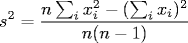We avoid this one-pass approach because it is numerically unstable. (See Exercise XYZ and XYZ.) This instability is most pronounced when the data has small variance but a large number of significant digits. In fact, it can cause the program to take the square root of a negative number! (See Exercise XYZ.) This subtlety surprises many uninitiated programmers. In fact, it even surprises some very experienced ones. Microsoft Excel versions 1.0 through 2002 implement the unstable one-pass algorithm in over a dozen of their statistical library functions. As a result, you may experience inaccurate results with no warning. These bugs were fixed with the release of Excel 2003.

Confidence intervals. Temperature in January vs. July.

Survey sampling. Census survey, temperature readings, election exit polls, quality control in manufacturing processes, auditing financial records, epidemiology, etc. Generally, newspapers report the result of some poll as something like 47% ± 3%. What does this really mean? Typically a 95% confidence interval is implicitly assumed. We assume the population consists of N elements, and we take a sample of size n, and sample i has an associated real value xi, which could represent weight or age. It could also represent 0 or 1 to denote whether some characteristic is present or absent (e.g., plan to vote for Kerry). The techniques from random sampling apply, except that we need to make a correction for the finite population size.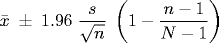When N is large relative to n (only a small fraction of the population is sampled), the finite population effects can be ignored.

Histogram. Program Histogram.java dynamically displays histogram as the data is accumulated.

Simple linear regression. In 1800 Giuseppe Piazzi discovered what appeared to be a new star and tracked its movement for 41 days before losing track of it due to bad weather. He was amazed since it moved in the opposite direction of the other stars. Carl Frederick Gauss used his newly invented method of least squares to predict where to find the star. Gauss became famous after the the star was located according to his prediction. As it turns out, the celestial body was an asteroid, the first one ever discovered. Now, the method of least squares is applied in many disciplines from psychology to epidemiology to physics. Gauss' famous computation involved predicting the location of an object using 6 variables. We first consider simple linear regression which involves only a single predictor variable x, and we model the response y = &beta0 + β1x. Given a sequence of n pairs of real numbers (xi, yi), we define the residual at xi to be ri = (yi - β0 - β1xi). The goal is to estimate values for the unobserved parameters β0 and β1 to make the residuals as small as possible. The method of least squares is to choose the parameters to minimize the sum of the squares of the residuals. Using elementary calculus, we can obtain the classic least squares estimates: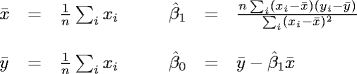Program LinearRegression.java reads in n measurements from standard input, plots them, and computes the line that best fits the data according to the least squares metric.

Assessing the fitted solution. To measure the goodness of fit, we can compute the coefficient of determination R2, which measure the fraction of variability in the data that can be explained by the variable x.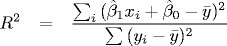We can also estimate the standard error, the standard error of the regression estimate for β0 and β1, and the 95% approximate confidence interval for the two unknown coefficients are.
Running time of algorithms. Take log of both sides. The slope is the exponent, the intercept is the constant.

Plot latitude vs. January temperature. Draw points within 2 standard deviations in black, between 2 and 3 in blue, above 3 in green. 18 of 19 outliers are in California or Oregon. The other is in Gunnison County, Colorado which is at very high altitude. Perhaps need to incorporate longitude and alititude into model...

Test for normality.

Multiple linear regression. Multiple linear regression generalizes simple linear regression by allowing several predictor variables instead of just one. We model the response y = β0 + β1x1 + ... + βpxp. Now, we have a sequence of n response values yi, and a sequence of n predictor vectors (xi1, xi2, ..., xip). The goal is to estimate the parameter vector (β0, ..., βp) so as to minimize the sum of the squared errors. In matrix notation, we have an overdetermined system of equations y = Xβ.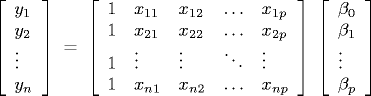Our goal is to find a vector β that minimizes ||Xβ - y||. Assuming X has full column rank, we can compute β by solving the normal equations XTXβ = XTy to compute our estimate of β. The simplest way to solve the normal equations is to explicitly compute A = XTX and b = XTy and solve the system of equations Ax = b using Gaussian elimination. A numerically stable algorithm for computing β is to compute QR factorization X = QR, then solve the triangular system Rβ = QTy via back substitution. This is exactly what Jama's solve method does when presented with an overdetermined system (assumes matrix has full column rank). Program MultipleLinearRegression.java is a straightforward implementation of this approach. See Exercise XYZ for an SVD-based method that works even if the system does not have full column rank).

An example. Weather data set and examples from this reference. Averag maximum daily temperatures at n = 1070 weather stations in US during March, 2001 Predictors = latitude (X1), longitude (X2), and elevation (X3). Model Y = 101 - 2 X1 + 0.3 X2 - 0.003 X3. Temp increases as longitude increases (west), but decreases as latitude increase (north) and alitude increase. Is effect of latitude on temperature greater in west or east? Plot scatterplot of temperature vs. latitude (divide at median longitude of 93 degrees) for March. Plot residuals vs. fitted values. Should not show any pattern.

Assesing the model. The error variance s2 is the sum of the squared error divided by the degrees of freedom (n - p - 1). The diagonal entries of the standard variance matrix is σ2(XTX)-1 estimate the variance of the parameter estimates.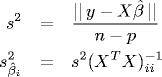Polynomial regression. predictor variables instead of just one. We model the response y = β0 + β1x1 + ... + βpxp. PolynomialRegression.java is a data type for performing polynomial regression.

## Discrete Fourier transform.

The discovery of efficient algorithms can have a profound social and cultural impact. The discrete Fourier transform is a method for decomposing a waveform of N samples (e.g., sound) into periodic components. The brute force solution takes time proportional to N2. At the age of 27, Freidrich Gauss proposed a method that requires only N log N steps, and he used it to analyze the periodic motion of the asteroid Ceres. This method was later rediscovered and popularized by Cooley and Tukey in 1965 after they described how to efficiently implement it on a digital computer. Their motivation was monitoring nuclear tests in the Soviet Union and tracking Soviet submarines. The FFT has become a cornerstone of signal processing, and is a crucial component of devices like DVD players, cell phones, and disk drives. It is also forms the foundation of many popular data formats including JPEG, MP3, and DivX. Also speech analysis, music synthesis, image processing. Doctors routinely use the FFT for medical imaging, including Magnetic Resonance Imaging (MRI), Magnetic Resonance Spectroscopy (MRS), Computer Assisted Tomography (CAT scans). Another important application is fast solutions to partial differential equations with periodic boundary conditions, most notably Poisson's equation and the nonlinear Schroedinger equation. Also used to simulate fractional Brownian motion. Without a fast way to solve to compute the DFT none of this would be possible. Charles van Loan writes "The FFT is one of the truly great computational developments of this [20th] century. It has changed the face of science and engineering so much that it is not an exaggeration to say that life as we know it would be very different without the FFT."

Fourier analysis is a methodology for approximating a function (signal) by a sum of sinusoidals (complex exponentials), each at a diffferent frequency. When using computers, we also assume that the the continuous function is approximated by a finite number points, sampled over a regular interval. Sinusoids play a crucial role in physics for describing oscillating systems, including simple harmonic motion. The human ear is a Fourier analyzer for sound. Roughly speaking, human hearing works by splitting a sound wave into sinusoidal components. Each frequency resonates at a different position in the basilar membrane, and these signals are delivered to the brain along the auditory nerve. One of the main applications of the DFT is to identify periodicities in data and their relative strengths, e.g., filtering out high frequency noise in acoustic data, isolating diurnal and annual cycles in weather, analyzing astronomical data, performing atmospheric imaging, and identifying seasonal trends in economic data.

The discrete Fourier transform (DFT) of a length N complex vector x is defined by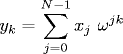where i is the square root of -1 and ω = e-2iπ/N is a principal Nth root of unity. We can also interpret the DFT as the matrix-vector product y = FN x, where FN is the N-by-N matrix whose jth row and kth column is ωjk. For example, when N = 4,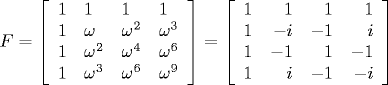We note that some authors define the Fourier matrix to be the conjugate of our Fourier matrix and normalize it by the factor 1 / sqrt(N) to make it unitary. Intuition: let xi be the samples of a signal over a time interval from 0 to T, and let fi be the DFT. Then f0 / n is an approximation of the average value of the signal over the interval. The modulus (absolute value) and argument (angle) of the complex number fj represent (one half) the amplitude and the phase of the signal component having frequency j / T for j = 1 to n/2 - 1.

Fast Fourier transform. It is straightforward to compute the DFT of a N-length vector either directly from the definition or via a dense matrix-vector multiplication. Both approaches take quadratic time. The Fast Fourier transform (FFT) is an ingenious method that computes the DFT in time proportional to N log N. It works by exploiting the symmetry of the Fourier matrix F. The crucial idea is to use properties of the nth roots of unity to relate the Fourier transform of a vector of size n to two Fourier transforms on vectors of size n/2.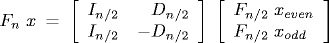where xeven denotes the vector of size n/2 consisting of x0, x2, ..., xn-2 and xodd denote the vector consisting of x1, x3, ..., xn-1, the matrix In/2 is the n/2-by-n/2 identity matrix and the matrix Dn/2 is the diagonal matrix whose kth diagonal entry is ωk. The radix 2 Cooley-Tukey FFT uses this recursive formula to compute the DFT in a divide-and-conquer style framework. Note that we have implicitly assumed that N is a power of 2. Program FFT.java is a bare bones implementation of this scheme. It relies on the Complex.java ADT developed in Section xyz. Program InplaceFFT.java is an in-place variant: it uses only O(1) extra memory.

Inverse FFT. The inverse DFT is defined as: The inverse of FN is the complex conjugate of itself, scaled down by a factor of N. Thus, to compute the inverse DFT of x: compute the DFT of the conjugate of x, take the conjugate of the result, and multiply each value by N.

Touch tone phones. Touch Tone ® telephones encode key presses as audio signals using a system called dual tone multi frequency (DTMF). Two audio frequncies are associated with each key press according to the table below

 Freqs 1209 Hz 1336 Hz 1477 Hz 1633Hz -------------------------------------------- 697 Hz 1 2 3 A 770 Hz 4 5 6 B 852 Hz 7 8 9 C 941 Hz * 0 # D 

For example, when the key 7 is pressed, the phone generates signals at frequencies 770 Hz and 1209 Hz, and sums them together. The frequencies should be within 1.5% of the proscribed values or the telephone company ignores it. The high frequencies must be at least as loud as the low frequency, but can be no more than 3 decibels louder.

notes

Commercial implementations. Because the FFTs great importance, there is a rich literature of efficient FFT algorithms, and there are many high optimized library implementations available (e.g., Matlab and the Fastest Fourier Transform in the West). Our implementation is a bare bones version that captures the most salients ideas, but it can be improved in a number of ways. For example, commercial implementations works for any N, not just powers of 2. If the input is real (instead of complex), they exploit additional symmetry and runs faster. They can also handle multidimensional FFTs. Our FFT implementation has a much higher memory footprint than required. With great care, it is even possible to do the FFT in-place, i.e., with no extra arrays other than x. Commericial FFT implementations also use iterative algorithms instead of recursion. This can make the code more efficient, but harder to understand. High performance computing machines have specialized vector processors, which can perform vector operations faster than an equivalent sequence of scalar operations. Although computational scientists often measure performance in terms of the number of flops (floating point operations), with the FFT, the number of mems (memory accesses) is also critical. Commercial FFT algorithms pay special attention to the costs associated with moving data around in memory. Parallel FFTs. Implemented in hardware.

Convolution. The convolution of two vectors is a third vector which represents an overlap between the two vectors. It arises in numerous applications: weighted moving average in statistics, shadows in optics, and echos in acoustics. Given two periodic signals a and b of length N, the circular convolution of a and b is defined by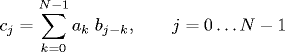and we use the notation c = a ⊗ b. The vector b is called the impulse response, filter, template, or point spread function. To see the importance of convolution, consider two degree N polynomials p(x) and q(x). Observe that the coefficients of r(x) = p(x) q(x) are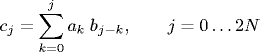obtained by convolving the coefficients of p with q, where p and q are padded with leading 0s to a length 2N. To facilitate computation, we also may pad with additional leading 0s to make its length a power of 2. This simulates the linear convolution since we don't want periodic boundary conditions.

A cornerstone result of Fourier analysis is the Convolution Theorem. It says that the DFT of a convolution of two vectors is the point-wise product of the DFT of the two vectors.

 DFTN(a ⊗ b) = DFTN(a) · DFTN(b) 

The Convolution Theorem is useful because the inverse DFT is easy to compute. This implies that we can compute the circular convolution (and hence polynomial multiplicaton) in N log N steps by taking three separate FFTs.

 a ⊗ b = (DFTN)-1 (DFTN(a) · DFTN(b)) 

This is amazing on two levels. First, it means that we can multiply two real (or complex) polynomials substantially faster than brute force. Second, the method relies on complex numbers even though multiplying two real polynomials appears to have nothing to do with imaginary numbers.

Matlab supplies a function conv that performs a linear convoultion of two vectors. However, their implementation takes quadratic time. In many applications, the vectors are large, say 1 million entries, and using this library function as a black box would be unacceptable. By exploiting our understanding of algorithms and complexity we can replace the library solution with an optimized one using the FFT!

 X = fft( [x zeros(1, length(y) - 1)] ) Y = fft( [y zeros(1, length(x) - 1)] ) c = ifft(X .* Y) 

As we have just witnessed, we can make dramatic performance improvements in computing the convolution, by first tranforming the data from the time domain to frequency domain. This same principle applies to related problems, including cross correlation, autocorrelation, polynomial multiplication, discrete sine and cosine transforms. It also means that we have fast matrix-vector multiplication algorithms for circulant matrices and Toeplitz matrices, which arise in numerical solutions to partial differential equations. f = signal, y = spectrum. f = impulse response, y = frequency response.

2D DFT. (exercise) Compute a 2D DFT of an N-by-N matrix by taking a DFT for each column, then taking a DFT of each row of the resulting values. N2 log N.

References: image compression, DFT book

#### Q + A

Q. Why is it called the Student's T distribution?

A. Discovered by an employee of Guinness brewing company named William Gosset in 1908, but Guinness did not allow him to publish under his own name, so he used "Student."

Q. Why minimize the sum of the squared errors instead of the sum of the absolute errors or some other measure?

A. Short answer: this is what scientists do in pratice. There are also some mathematical justifications. The Gauss-Markov theorem says that if you have a linear model in which the errors have zero mean, equal variance, and are uncorrelated, then the least squares estimates of a and b (the ones that minimize the sum of the squared error) have the smallest variance among all unbiased estimates of a and b. If in addition we assume that the errors are independent and normally distributed, then we can derive 95% or 99% confidence intervals...

Q. Where can I get a charting library?

A. Check out JFreeChart. Here are some instructions on using it. Or see the Scientific Graphics Toolkit for creating interactive, publication quality graphics of scientific data.

#### Exercises

1. Baseball statistics.. Do some analysis of baseball statistics.
2. Histogram. Modify Histogram.java so that you do not have to input the range ahead of time.
3. Histogram. Modify Histogram.java so that it has 10 buckets.
4. Pie chart.
5. Stem-and-leaf plot.
6. Simple linear regression. Modify program LinearRegression.java to plot and scale the data. Again, we are careful to choose a stable algorithm instead of a slightly simpler one-pass alternative.

#### Creative Exercises

1. One-pass algorithm. Write a program OnePass.java that computes the sample mean and variance in one pass (instead of two) using the alternate textbook formula.
 sum = x1 + ... + xN sum2 = x1x1 + ... + xNxN σ = sqrt ( (N sum2 - sum * sum) / (N*(N-1)) ) 

Verify that it is not numerically stable by plugging in n = 3, x1 = 1000000000, x2 = 1000000001, and x3 = 1000000002. The one pass algorithm gives a variance of 0, but the true answer is 1. Also, verify that it can lead to taking the square root of a negative number by plugging in an input with n = 2, x1 = 0.5000000000000002 and x2 = 0.5000000000000001. Compare with Average.java.

2. Sample variance. Implement the following stable, one-pass algorithm for computing the sample variance. Verify that the formula is correct.
 m1 = x1, mk = mk-1 + (xk - mk-1)/k s1 = 0, sk = sk-1 + ((k-1)/k)(xk - mk-1)2 μ = mN σ = sqrt(sN/(N-1)) 
3. Normal quantile plot. To test whether a given set of data follows a normal distribution, create a normal quantile plot of the data and check whether the points lie on (or close to) a straight line. To create a normal quantile plot, sort the N data points. Then plot the ith data point against Φ-1(i / N).
4. Diamond 3D graphs. Write a program to read in a set of three dimensional data and plot a diamond graph of the data like the one below. Diamond graphs have several advantages over 3D bar charts.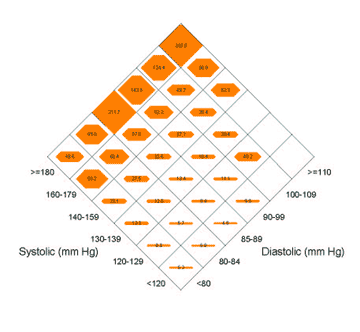5. Polynomial curve fitting. Suppose we have a set of N observations (xi, yi) and we want to model the data using a low degree polynomial.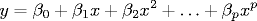Empirically collect n samples: (xi, yi). In matrix notation, our least squares problem is: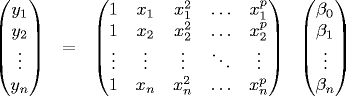The Matrix X is called the Vandermonde matrix and has full column rank if n ≥ p and the xi are distinct. Our problem is a special case of general linear regression. The solution vector β are the coefficients of the best fit degree p polynomial.

6. Rank deficient linear regression. Better method: use SVD. Better numerical stability properties. Works even if A doesn't have full rank. Compute the skinny SVD: A = UrΣrVrT. Here r is the rank of A, Ur, Σr, and Vr are the first r columns of U, Σ, and V, respectively. The pseduoinverse A = Urr)-1VrT and the least squares estimate x* = Ab. The pseudoinverse nicely generalizes the matrix inverse: if A is square and invertible then A = A-1. If A is skinny and has full rank then A = (ATA)-1AT. To compute Ab, don't explictly form the pseudoinverse. Instead, compute v = VTb, w = Σ-1u, x* = Uw. Note that Σ-1 is easy to compute since Σ is diagonal.

In Matlab, pinv(A) gives pseudoinverse and svd(A, 0) gives thin SVD for skinny matrices (but not fat ones!)

7. Underdetermined system. In data fitting applications, the system of equations is typically overdetermined and A is skinny. In control systems, we have have an underdetermined system of equations and the goal is to find an x* that solves Ax* = b such that the norm of x* is minimized. Again, the SVD comes to the rescue. If A has full column rank, then Ab is such a solution.
8. Polynomial multiplication. Give two polynomials of degree m and n, describe how to compute their product in time O(m log n).

Clustering. Evolutionary trees in biology, marketing research in business, classifying painters and musicians in liberal arts, classifying survey responses in sociology, [reference: Guy Bleloch]